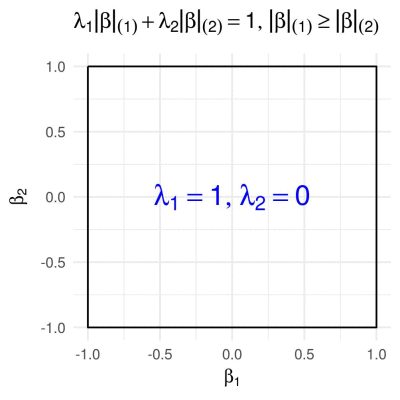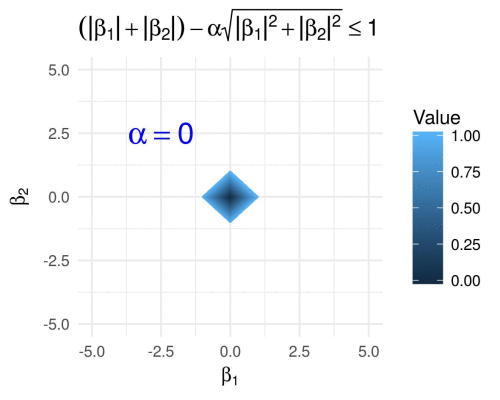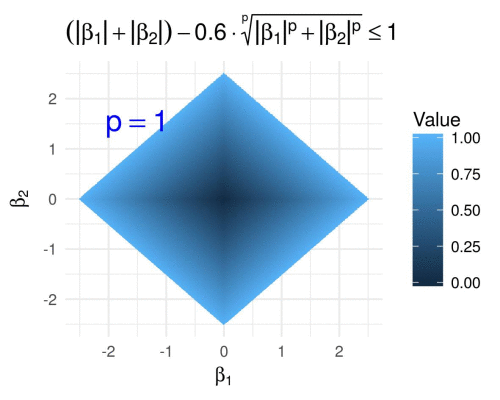# Contours of statistical penalty functions as GIF images

March 17, 2017
By

Want to share your content on R-bloggers? click here if you have a blog, or here if you don't.

Many statistical modeling problems reduce to a minimization problem of the general form:

or

where is some type of loss function, denotes the data, and is a penalty, also referred to by other names, such as “regularization term” (problems (1) and (2-3) are often equivalent by the way). Of course both, and , may depend on further parameters.

There are multiple reasons why it can be helpful to check out the contours of such penalty functions :

1. When is two-dimensional, the solution of problem (2-3) can be found by simply taking a look at the contours of and .
2. That builds intuition for what happens in more than two dimensions, and in other more general cases.
3. From a Bayesian point of view, problem (1) can often be interpreted as an MAP estimator, in which case the contours of are also contours of the prior distribution of .

Therefore, it is meaningful to visualize the set of points that maps onto the unit ball in , i.e., the set

Below you see GIF images of such sets for various penalty functions in 2D, capturing the effect of varying certain parameters in . The covered penalty functions include the family of -norms, the elastic net penalty, the fused penalty, the sorted norm, and several others.

:white_check_mark: R code to reproduce the GIFs is provided.

## p-norms in 2D

First we consider the -norm,

with a varying parameter (which actually isn’t a proper norm for ). Many statistical methods, such as LASSO (Tibshirani 1996) and Ridge Regression (Hoerl and Kennard 1970), employ -norm penalties. To find all on the boundary of the 2D unit -norm ball, given (the first entry of ), is easily obtained as## Elastic net penalty in 2D

The elastic net penalty can be written in the form

for . It is quite popular with a variety of regression-based methods (such as the Elastic Net, of course). We obtain the corresponding 2D unit “ball”, by calculating from a given as## Fused penalty in 2D

The fused penalty can be written in the form

It encourages neighboring coefficients to have similar values, and is utilized by the fused LASSO (Tibshirani et. al. 2005) and similar methods.(Here I have simply evaluated the fused penalty function on a grid of points in , because figuring out equations in parametric form for the above polygons was too painful for my taste… :stuck_out_tongue:)

## Sorted L1 penalty in 2D

The Sorted penalty is used in a number of regression-based methods, such as SLOPE (Bogdan et. al. 2015) and OSCAR (Bondell and Reich 2008). It has the form

where are the absolute values of the entries of arranged in a decreasing order. In 2D this reduces to## Difference of p-norms

It holds that

or more generally, for all -norms it holds that

Thus, it is meaningful to define a penalty function of the form

for , which results in the following.We visualize the same for varying fixing , i.e., we define

and we obtain the following GIF.## Hyperbolic tangent penalty in 2D

The hyperbolic tangent penalty, which is for example used in the method of variable selection via subtle uprooting (Su, 2015), has the form